• 使用python读取excel文件
读入文件
import xlrd
filename = ('filename.xls')
file = xlrd.open_workbook(filename)

读取excel文件中的表格及表格内容
(表格中的sheet名为数字)
sheet={}
for i in range(1,7,1):
sheetname=str(i)
sheet[i]=result.sheet_by_name(sheetname)
print(sheet.cell(0,0).value) # 读取sheet1(0,0)单元格的值验证

获取表格的行数和列数
sheet1 = file.sheet_by_name('sheet1')
# 获取表的行数
rows = sheet1.nrows
# 获取表的列数
cols = sheet1.ncols
print("sheet1 行: %d, 列: %d" % (rows, cols))

使用cell.value获取的单元格的值类型为cell，如果需要计算，需要用float()函数转换为浮点数


展开全文• ## Python读取Excel文件

万次阅读 多人点赞 2018-03-06 14:22:16
Python读取excel文件内容十分方便，为以下几个步骤： （1）安装python官方Excel库–&gt;xlrd （2）获取Excel文件位置并读取 （3）读取sheet （4）读取指定rows和cols内容 安装xlrd 2.读取Excel文件...
Python读取excel文件内容十分方便，为以下几个步骤：
（1）安装python官方Excel库–>xlrd
（2）获取Excel文件位置并读取
（3）读取sheet
（4）读取指定rows和cols内容

安装xlrd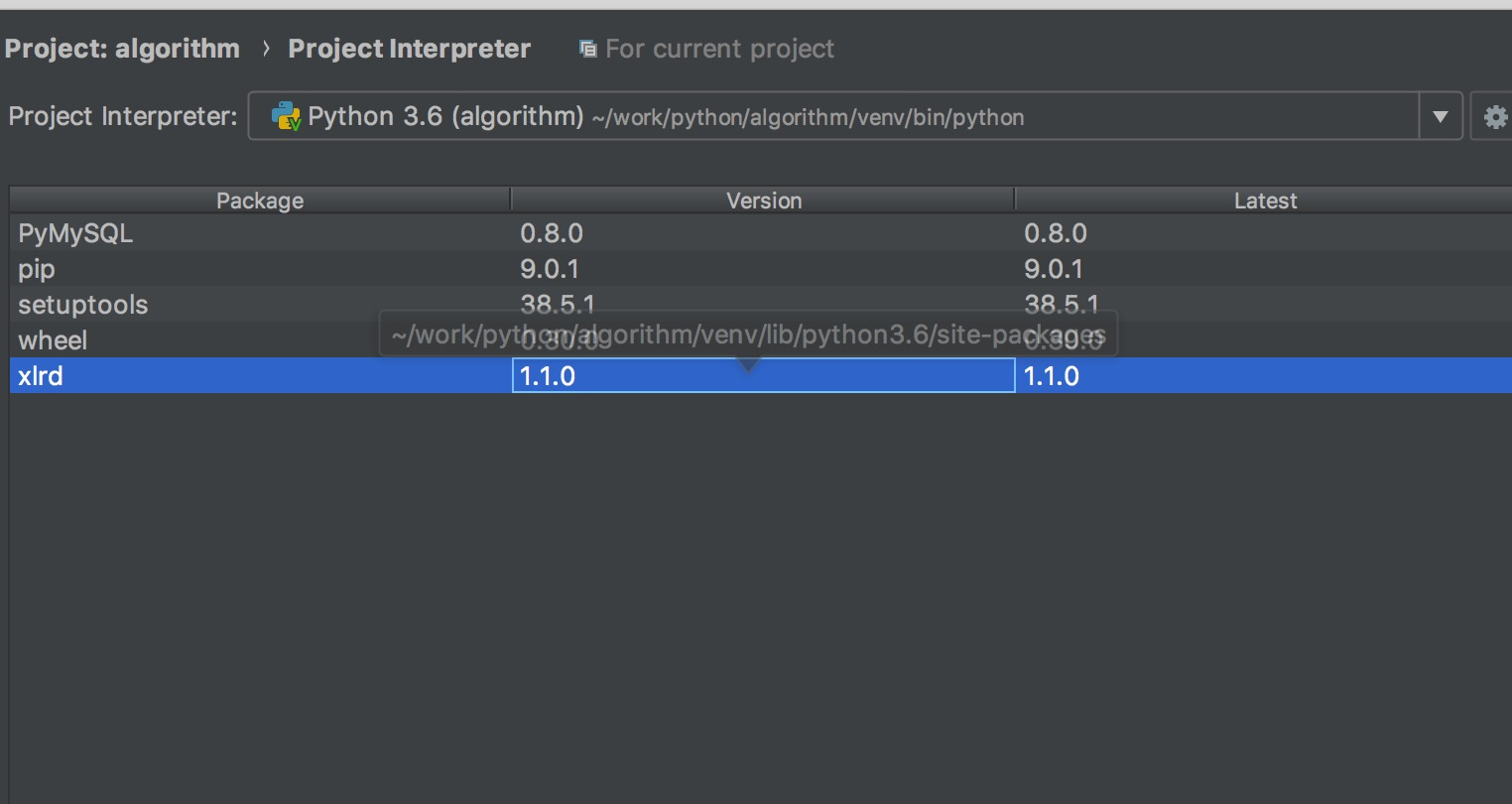2.读取Excel文件位置

    def read_file(file_url):
try:
data = xlrd.open_workbook(file_url)
return data
except Exception as e:
print(str(e))

3.读取sheet

def filter_excel(workbook, column_name=0, by_name='Sheet0'):
"""

:param workbook:
:param column_name:
:param by_name: 对应的Sheet页
:return:
"""
table = workbook.sheet_by_name(by_name)  # 获得表格
total_rows = table.nrows  # 拿到总共行数
columns = table.row_values(column_name)  # 某一行数据 ['姓名', '用户名', '联系方式', '密码']
excel_list = []
for one_row in range(1, total_rows):  # 也就是从Excel第二行开始，第一行表头不算

row = table.row_values(one_row)
if row:
row_object = {}
for i in range(0, len(columns)):
row_object[key] = row[i]  # 表头与数据对应

excel_list.append(row_object)

return excel_list

workbook.sheet_by_name(”Sheet0”)
根据Sheet的名称获取对应的Sheet，也能根据sheet_by_index,详细见API: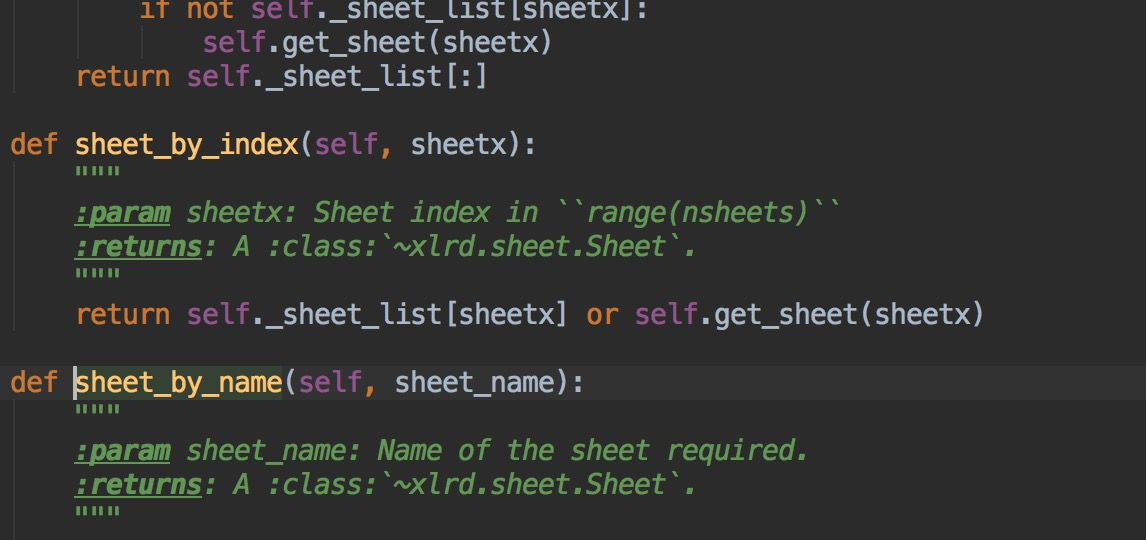4.读取指定rows和cols内容


columns = table.row_values(0)  # 某一行数据 ['姓名', '用户名', '联系方式', '密码']

第0行为表格头部：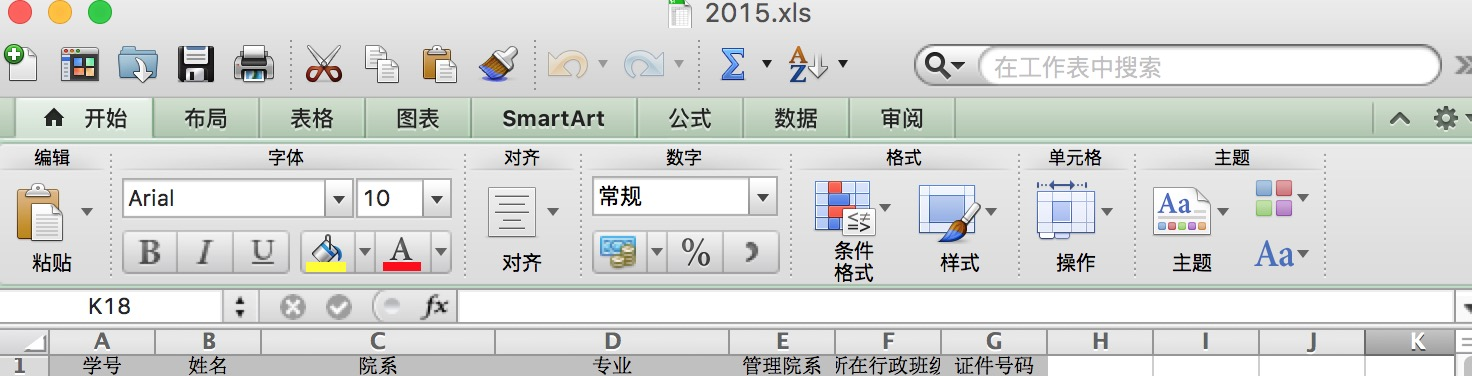excel_list = []
total_rows = table.nrows  # 拿到总共行数
for one_row in range(1, total_rows):  # 也就是从Excel第二行开始，第一行表头不算

row = table.row_values(one_row)
if row:
row_object = {}
for i in range(0, len(columns)):
row_object[key] = row[i]  # 表头与数据对应

excel_list.append(row_object)


读取每一行数据。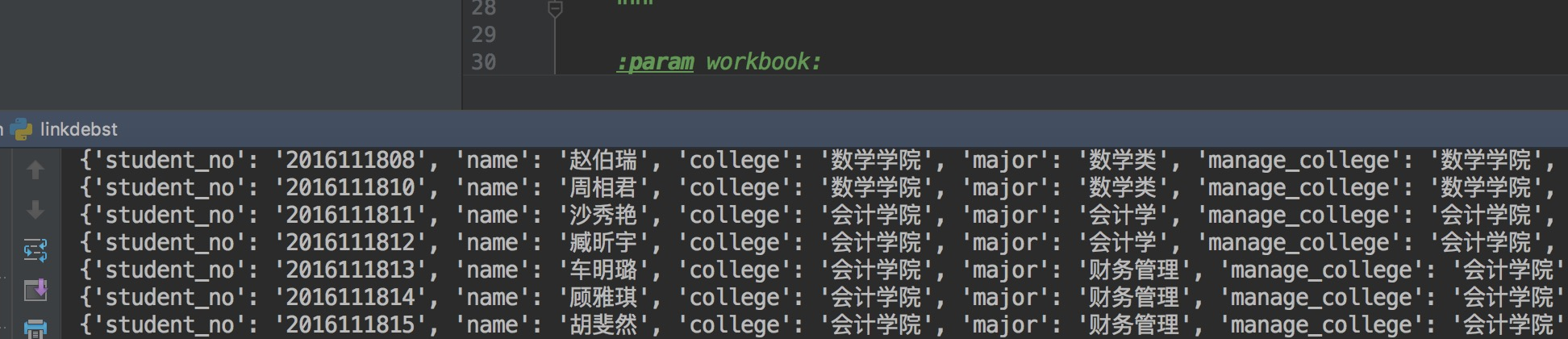展开全文Excel Sheet
• 现在大部分数据都是存储在excel中或直接调取数据库，作为刚刚自学Python的小白来说怎么读取文件还是比较懵的，现在对Python读取excel文件进行了一些整理： #coding=utf-8 #cmd中进行安装xlrd库 pip install ...

现在大部分数据都是存储在excel中或直接调取数据库，作为刚刚自学Python的小白来说怎么读取文件还是比较懵的，现在对Python读取excel文件进行了一些整理：

#coding=utf-8
#cmd中进行安装xlrd库
pip install xlrd
import xlrd
#文件路径，要用/而不是\
file_path = r'C:/Users/mingli.zhao/Desktop/七天.xlsx'
#中文转码
#file_path = file_path.decode('utf-8')
#获取数据
data = xlrd.open_workbook(file_path)
#获取sheet
table = data.sheet_by_name('Sheet1')
#获取总行数
nrows = table.nrows
#获取总列数
ncols = table.ncols
#获取第一行的数值
table.row_values(1)
#获取第一列的数值
table.col_values(1)
#获取第二行第一列单元格的数值
cell_value = table.cell(1,0).value

转载于:https://www.cnblogs.com/Doers/p/9342185.html
展开全文• python 读取excel数据 pip install xlrd import xlrd def read_excel(): ... 读取Excel文件 :return: """ # 打开excel文件进行数据提取 excel_file = xlrd.open_workbook('demo/test.xls...
python 读取excel数据

pip install xlrd

import xlrd

"""
读取Excel文件
:return:
"""
# 打开excel文件进行数据提取
excel_file = xlrd.open_workbook('demo/test.xlsx')

# 获取Excel文件sheet名
excel_file.sheet_names()
print(excel_file.sheet_names())
# 有多个sheet时需要指定读取目标sheet
sheet1_name = excel_file.sheet_names()
print(sheet1_name)
# 获取sheet1内容
# 通过index读取
# sheet1_info = excel_file.sheet_by_index(0)
# 通过对应的名字读取
sheet1_info = excel_file.sheet_by_name('Sheet1')

# 读取Excel文件所有数据
values = []
for i in range(sheet1_info.nrows):
print(sheet1_info.row_values(i))
values.append(sheet1_info.row_values(i))

# 获取指定行和列数据
rows = sheet1_info.row_values(1)
cols = sheet1_info.col_values(2)
# 获取表格里的内容，三种方式
print(sheet1_info.cell(1, 2).value)  # 获取第二行第三列数据
print(sheet1_info.cell_value(1, 0))
print(sheet1_info.row(1).value)

print(rows, cols)

test.xlsx文件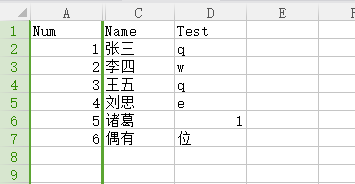转载于:https://www.cnblogs.com/CesareZhang/p/11049553.html
展开全文• 在使用python读取Excel文件中的时间格式，碰到的时间格式转换问题：读取这样的表格：输出这样的数据结果：然而这样的结果却不是我们想要的，我们需要的是这样的结果:1、安装python官方库---datetime引入datetime库...
• 1. excel文件只包含一个sheet表 import xlrd """读取包含耽搁sheet的excel数据""" workbook = xlrd.open_workbook("test.xlsx") # 打开工作表 sheet0 = workbook.sheet_by_index(0) # 获取工作簿 nrows = sheet0.n...自然语言处理
• def read_excel_model(file, modelBase=None, dbFields=None, rackhdRegion=... 读取excel内容，自动转换为model实体，如果读取为float自动转为int :param file: 文件路径: :param modelBase: 实体类: :param d...
• 这是一个python读取excel文件例子，里面配有python文件、python文件生成的exe，并有MFC界面调取exe文件
• python 读取excel文件生成sql文件实例详解学了python这么久，总算是在工作中用到一次。这次是为了从excel文件中读取数据然后写入到数据库中。这个逻辑用java来写的话就太重了，所以这次考虑通过python脚本来实现。在......

# python读取excel文件python 订阅